Learning Library

Score some points with these basketball math worksheets, covering addition, subtraction, multiplication, and division.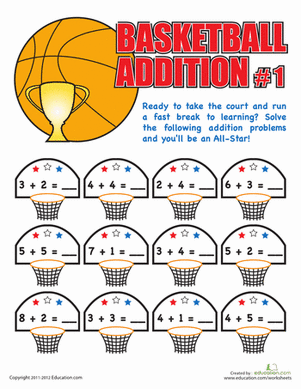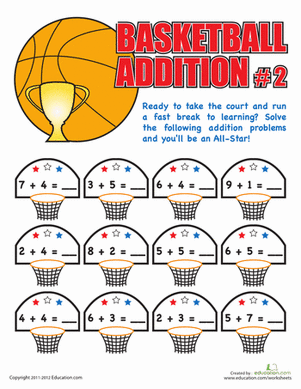Here's a supplemental math activity that beats boring textbook math. Your child will work on his addition facts, practicing to become an addition all star!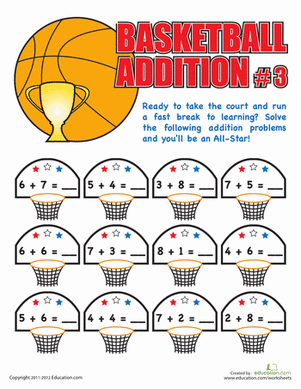How fast can your child do his addition facts? Test his math skills with a fun basketball math sheet, and he'll practice doing one-digit addition equations.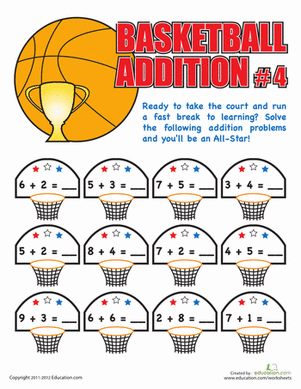Is your child an addition all star? Test his math handling skills with a fun basketball math sheet, where he'll work on one-digit addition facts.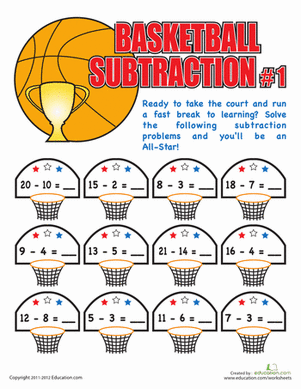Bring your a-game to this subtraction worksheet, and see how many subtraction problems you can solve!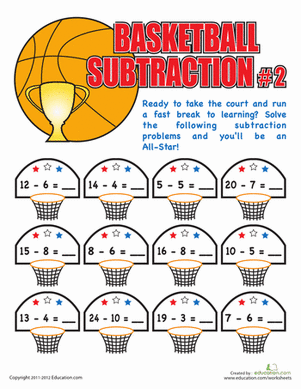How many subtraction shots can you make? Challenge your first grader's subtraction skills with a fun basketball themed math sheet.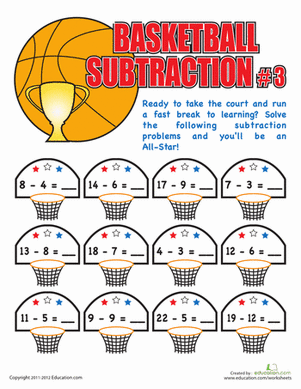If your child is a math all-star, give him a fun supplemental sheet where he'll get to show off his subtraction skills!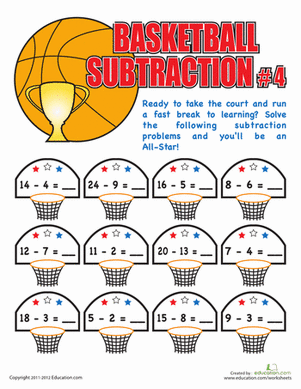See how savvy your child's subtraction skills are with a fun basketball math sheet. She'll get to do a few one and two digit subtraction problems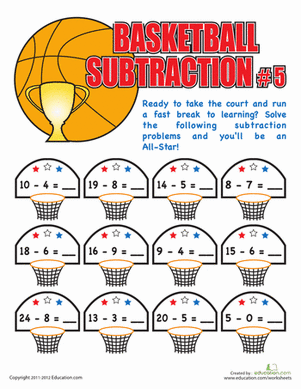Give your all-star math student a fun challenge with this basketball subtraction sheet! She'll work on one and two digit subtraction problems.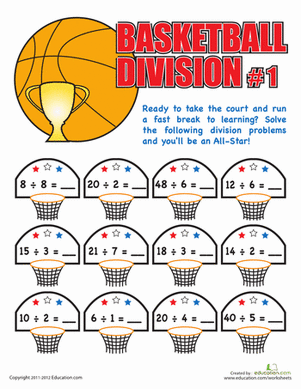Is your child a times tables all-star? Give her a fun challenge with a few division problems, a great way to review the times tables.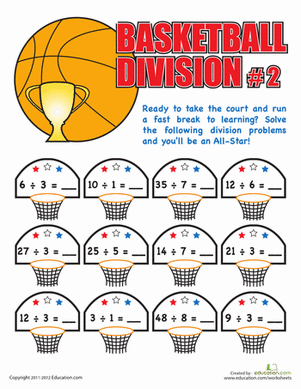Put your child's math skills to the test with a fun division worksheet. He'll practice some simple division problems, a great way to review times tables!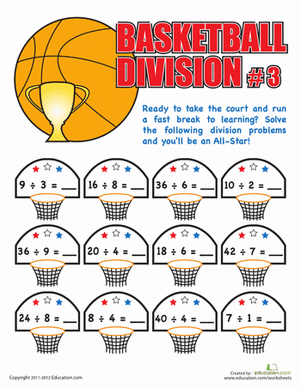Take a break from boring textbook math, and practice division with a basketball math sheet! Your child will work on simple division.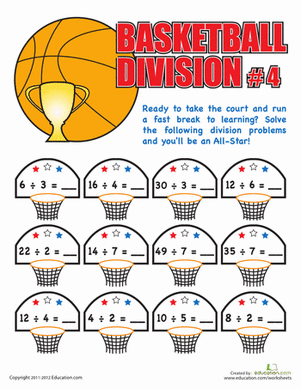Get your child pumped up for math practice with a fun basketball division sheet! He'll try to complete all the division equations as quick as possible.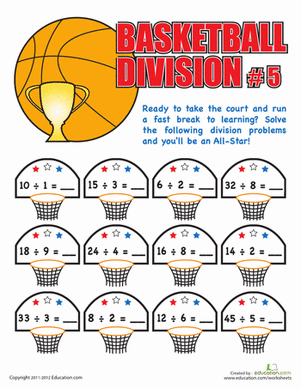Is your math whiz acing his times tables? Give him a great challenge with this basketball division sheet, where he'll practice division facts.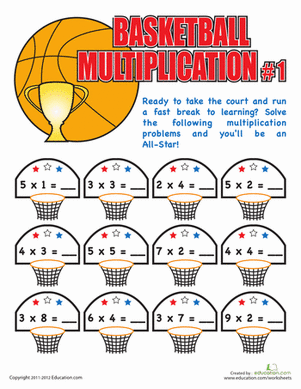Run circles around math practice with a fun multiplication sheet! Your math whiz can work on her times tables, basketball style.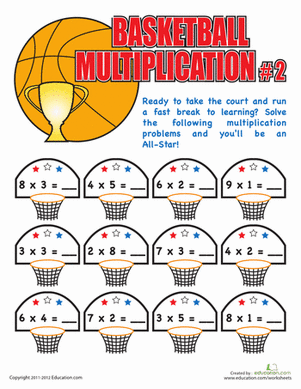Think your child's got a handle on her times tables? Give her a fun challenge with this basketball multiplication sheet.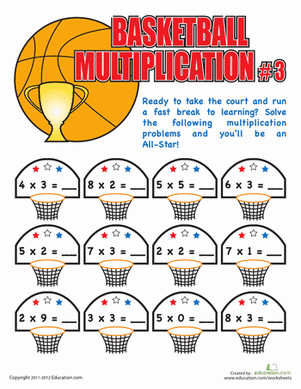Give your all star math student a fun challenge with this basketball math sheet! She'll get to review her times tables, perfect practice for a quiz.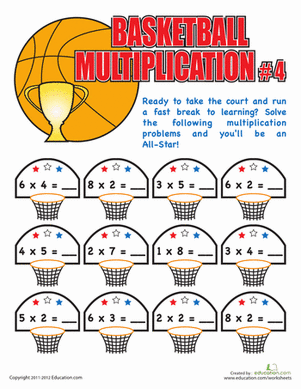Math maniacs, sneak in some multiplication practice with a fun basketball math sheet. Your child will get a great review of times tables.

Create new collection

0

### New Collection>

0 items

What could we do to improve Education.com?